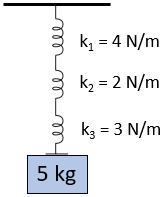## Series of Springs

Consider three springs in series are attached to a fixed point on a ceiling as shown in the figure. If a 5 kg mass is attached at the end, how much will the springs stretch in total?Hint
The force in a spring is:
$$F_s=k\delta$$$where $$k$$ is the spring constant, and $$\delta$$ is the change in spring length from the un-deformed spring length. Hint 2 The spring constant for springs in series: $$\frac{1}{k_{eq}}=\sum_{i}\frac{1}{k_i}$$$
First, let’s determine the equivalent spring constant for several springs in series:
$$\frac{1}{k_{eq}}=\sum_{i}\frac{1}{k_i}$$$Since the problem statement has three springs in series: $$\frac{1}{k_{eq}}=\frac{1}{k_1}+\frac{1}{k_2}+\frac{1}{k_3}=\frac{1}{4N/m}+\frac{1}{2N/m}+\frac{1}{3N/m}$$$
$$\frac{1}{k_{eq}}=\frac{3}{12N/m}+\frac{6}{12N/m}+\frac{4}{12N/m}=\frac{13m}{12N}$$$$$\frac{1}{\frac{1}{k_{eq}}}=\frac{1}{\frac{13m}{12N}}$$$
$$k_{eq}=\frac{12}{13}\:N/m$$$A spring’s deflection and force are related by: $$F_s=k_{eq}\delta$$$
where $$F_s$$ is the forced applied to the spring, $$k_{eq}$$ is the equivalent spring constant, and $$\delta$$ is the change in spring length from the un-deformed spring length.
Remember, $$Force = mass \times acceleration$$ . Therefore, the spring will stretch a total of:
$$\delta=\frac{F_s}{k_{eq}}=\frac{13m}{12N}\times (5kg)(9.8m/s^2)$$$$$=\frac{13m}{12N}\times 49N=53.1\:m$$$
53.1 m